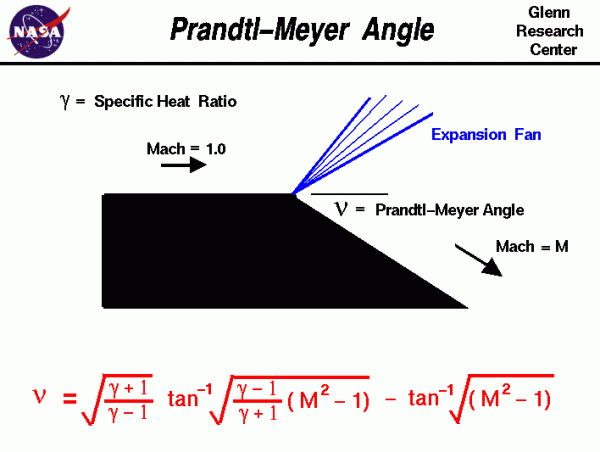# Prandtl-Meyer AngleAs an object moves through a gas, the gas molecules are deflected around the object. If the speed of the object is much less than the speed of sound of the gas, the density of the gas remains constant, and the flow of gas can be described by conserving momentum and energy. As the speed of the objects increases towards the speed of sound, we must consider compressibility effects on the gas. The density of the gas varies locally as the gas is compressed by the object. When an object moves faster than the speed of sound, and there is an abrupt decrease in the flow area, shock waves are generated. If the flow area increases, however, a different flow phenomenon is observed. If the increase is abrupt, we encounter a centered expansion fan.

There are some marked differences between shock waves and expansion fans. Across a shock wave, the Mach number decreases, the static pressure increases, and there is a loss of total pressure because the process is irreversible. Through an expansion fan, the Mach number increases, the static pressure decreases, and the total pressure remains constant. Expansion fans are isentropic.

## Prandtl-Meyer Function

The calculation of the expansion fan involves the use of the Prandtl-Meyer function. This function is derived from conservation of mass, momentum, and energy for very small (differential) deflections. The Prandtl-Meyer function is denoted by the Greek letter nu ($$\bf \nu$$) on the slide and is a function of the Mach number M and the ratio of specific heats gam ($$\bf \gamma$$) of the gas:

$$\LARGE \nu=\sqrt{\frac{\gamma+1}{\gamma-1}}\tan^{-1}\sqrt{(\gamma-1)(M^{2}-1)}-\tan^{-1}\sqrt{M^{2}-1}$$

where tan-1 is the trigonometric inverse tangent function. The meaning of tan-1 can be explained by these two equations:

$$\LARGE \tan^{-1}a=b$$

$$\LARGE \tan b=a$$

As mentioned above, the Mach number of a supersonic flow increases through an expansion fan. The amount of the increase depends on the incoming Mach number and the angle of the expansion. The physical interpretation of the Prandtl-Meyer function is that it is the angle through which you must expand a sonic (M=1) flow to obtain a given Mach number. The value of the Prandtl-Meyer function is therefore called the Prandtl-Meyer angle.

## Interactive Simulation

Here is a JavaScript program which solves for the Prandtl-Meyer angle:

Please note: the simulation below is best viewed on a desktop computer. It may take a few minutes for the simulation to load.

Note 2: Putting in Gamma >= 1.45 and Prandtl-Meyer Angle>=1.45 causes the program to crash, we are working on a fix for this issue.

## General Instructions

Select an input variable by using the choice button labeled Input Variable. Next to the selection, you then type in the value of the selected variable. When you hit the COMPUTE button, the output values change. The default input variable is the Mach number, and by varying Mach number you can see the effect on Prandtl-Meyer angle. You can also select Prandtl-Meyer angle as an input and see its effect on the flow variables downstream of the turning.

Provide feedback